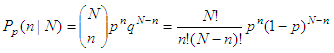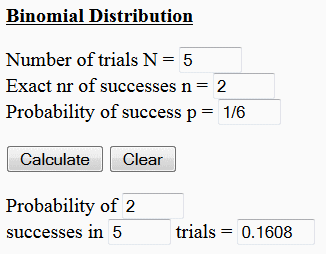Binomial Distribution Calculator

The Binomial Distribution gives the discrete probability distribution Pp(n|N) of obtaining exactly n successes out of N Bernoulli trials (where the result of each Bernoulli trial is true with probability p and false with probability q = 1-p). The binomial distribution is given by this formulaThe binomial distribution calculator below asks to enter three parameters: the number of trials (N), the exact number of successes (n), and the probability of success (p).

Example:

What is the probability that in five rolls of a fair die, a one appears twice?

N = 5
n = 2
p = 1/6 = 0.16666

This is an image of how you can enter the data into the calulator:Please, enable JavaScript codes on this page, otherwise the calculator will not work.

 Binomial Distribution Number of trials N = Exact nr of successes n = Probability of success p = Probability of successes in trials =

From 'Binomial Distribution Calculator' to home

From 'Binomial Distribution' to 'Online Calculators'

 Top Binomial Distribution Code in Matlab